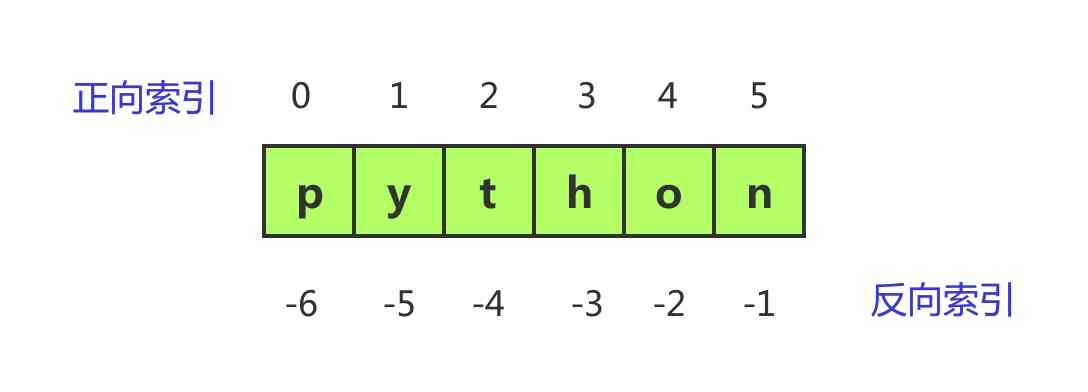# python字符串(str)概念

## 1. 字符串的创建

``````str_1 = 'python'
str_2 = "python"
str_3 = """python"""

print(str_1, type(str_1))
print(str_2, type(str_2))
print(str_3, type(str_3))
``````

``````python <class 'str'>
python <class 'str'>
python <class 'str'>
``````

``````>>> a = 'I'
>>> b = 'like'
>>> c = 'python'
>>> d = a + ' ' + b + ' ' + c
>>> d
'I like python'
>>> len(d)
13
``````

## 2. 索引的概念

python中，字符串是字符的有序集合。这里，你主要关注有序二字。

``````'python'
'443'
'*&^%\$'
````````````>>> a = 'python'
>>> a
'p'
>>> a[-2]
'o'
>>> a[1:3]
'yt'
>>> a
Traceback (most recent call last):
File "<stdin>", line 1, in <module>
IndexError: string index out of range
``````

a[1:3]，表示范围索引1到索引3这个范围内的字符，得到的结果是'yt'， 这就是切片操作，关于切片操作会有专门的文章讲解。

## 3. 字符串的运算

+ 字符串连接
* 重复字符串
[] 通过索引访问指定索引的字符
[ : ] 切片操作，截取字符串指定范围
in 成员运算符 - 如果字符串中包含给定的字符返回 True
not in 成员运算符 - 如果字符串中不包含给定的字符返回 True
% 格式字符串

``````>>> a = 'py'
>>> b = 'python'
>>> a + b
'pypython'
>>> a*3
'pypypy'
>>> b
'h'
>>> b[0:2]
'py'
>>> a in b
True
>>> b not in b
False
``````QQ交流群: 211426309